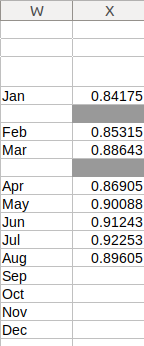# Find value in last cell in column

In the below table (part of a greater sheet) I have a table of ECB monthly EUR/GBP closing rates. The two blanks are in respect of two additional occasions in a 28 day cycle and contain no data and are thus disregarded. The array in the example comprises cells in the range X4:X17.What I am seeking to do in another cell, is enter the most recent rate (in the example given 0.89605).

Currently, I just re-enter the data (overwriting what is already there in the reference cell) but I am certain there is a simple formula to achieve this objective.

I have tried VLOOKUP(), COUNTIFS(), INDEX() in various forms but with no success.

I’m certain there is a simple solution to this but just now, it evades me.

Can anyone propose a suitable, simple solution to return the value in the last occupied cell in a column of 14 data elements?

What about `=INDIRECT("X"&SUMPRODUCT(MAX(NOT(ISBLANK(X:X))*ROW(X:X))))`?

Not sure how that works but it returns a value of 23154.74. How does that relate to 0.89605 (the expected result)?

… then far below your cell with value `0.89605` there is another cell having value `23154.74`. The formula is a full replacement for my second formula and according to your comment to my answer adaption to `=INDIRECT("X"&SUMPRODUCT(MAX(NOT(ISBLANK(X4:X17))*ROW(X4:X17))))` will produce the same result.

You’re right! Hadn’t noticed that. Amended to your new version and that works. Thanks.

Hello,

try the following formula:

`=OFFSET(\$X\$1;SUMPRODUCT(MAX((X1:X1000<>"")*(ROW(X1:X1000))))-1;0;1;1)`, where you may need to adapt `1000` in `X1000` to the real maximum number to appear in your sheet. You may also use:

`=OFFSET(\$X\$1;SUMPRODUCT(MAX((X:X<>"")*(ROW(X:X))))-1;0;1;1)`, but since this involves whole columns it may cause performance problems (if something doesn’t work as it should), hence I generally try to avoid this.

Hope that helps.

Thanks - the first example works perfectly when adjusted to within the range X4 to X17